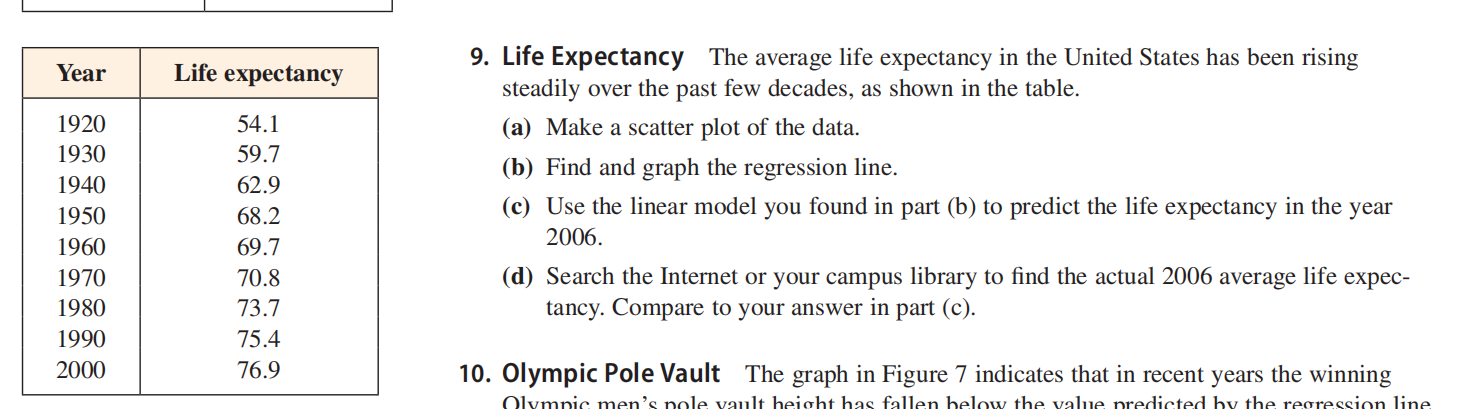### ¿Todavía tienes preguntas de matemáticas?

Pregunte a nuestros tutores expertos
Algebra
PreguntaLife Expectancy The average life expectancy in the United States has been rising steadily over the past few decades, as shown in the table.

(a) Make a scatter plot of the data.

(b) Find and graph the regression line.

(c) Use the linear model you found in part (b) to predict the life expectancy in the year $$2006 .$$

(d) Search the Internet or your campus library to find the actual $$2006$$ average life expec- tancy. Compare to your answer in part (c).

$$\left. \begin{array} { l } { \text { 9. (a) } } \\ { \left. \begin{array} { l l } { \text { (b) } y = 0.2708 x - 462.9 } & { \text { (c) } 80.4 \text { years } } \end{array} \right. } \end{array} \right.$$